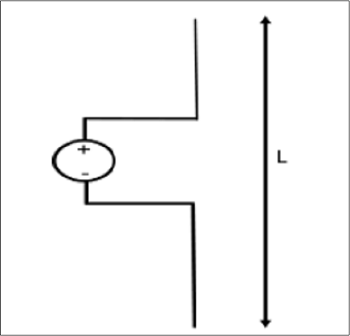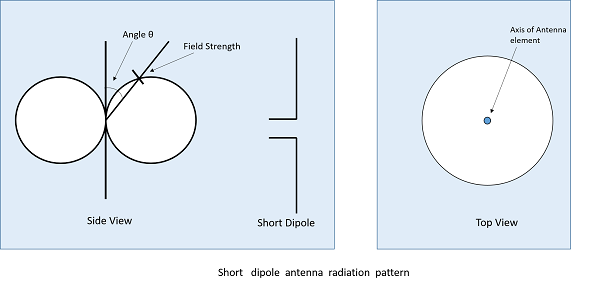# Antenna Theory - Short Dipole

A short dipole is a simple wire antenna. One end of it is open-circuited and the other end is fed with AC source. This dipole got its name because of its length.

### Frequency range

The range of frequency in which short dipole operates is around 3KHz to 30MHz. This is mostly used in low frequency receivers.

## Construction & Working of Short Dipole

The Short dipole is the dipole antenna having the length of its wire shorter than the wavelength. A voltage source is connected at one end while a dipole shape is made, i.e., the lines are terminated at the other end.The circuit diagram of a short dipole with length L is shown. The actual size of the antenna does not matter. The wire that leads to the antenna must be less than one-tenth of the wavelength. That is

$$L < \frac{\lambda}{10}$$

Where

• L is the length of the wire of the short dipole.

• λ is the wavelength.

Another type of short dipole is infinitesimal dipole, whose length is far less than its wave length. Its constructiion is similar to it, but uses a capacitor plate.

## Infinitesimal Dipole

A dipole whose length is far less than wavelength is infitesimal dipole. This antenna is actually impractical. Here, the length of the dipole is less than even fiftith part of the wavelength.

The length of the dipole, Δl << λ. Where, λ is the wavelength.

$$\Delta l = \frac{\lambda}{50}$$

Hence, this is the infinitely small dipole, as the name implies.

As the length of these dipoles is very small, the current flow in the wire will be dI. These wires are generally used with capacitor plates on both sides, where low mutual coupling is needed. Because of the capacitor plates, we can say that uniform distribution of current is present. Hence the current is not zero here.

The capacitor plates can be simply conductors or the wire equivalents. The fields radiated by the radial currents tend to cancel each other in the far field so that the far fields of the capacitor plate antenna can be approximated by the infinitesimal dipole.

The radiation pattern of a short dipole and infinitesimal dipole is similar to a half wave dipole. If the dipole is vertical, the pattern will be circular. The radiation pattern is in the shape of “figure of eight” pattern, when viewed in two-dimensional pattern.

The following figure shows the radiation pattern of a short dipole antenna, which is in omni-directional pattern.The following are the advantages of short dipole antenna −

• Ease of construction, due to small size

• Power dissipation efficiency is higher

The following are the disadvantages of short dipole antenna −

• High resistive losses
• High power dissipation
• Low Signal-to-noise ratio